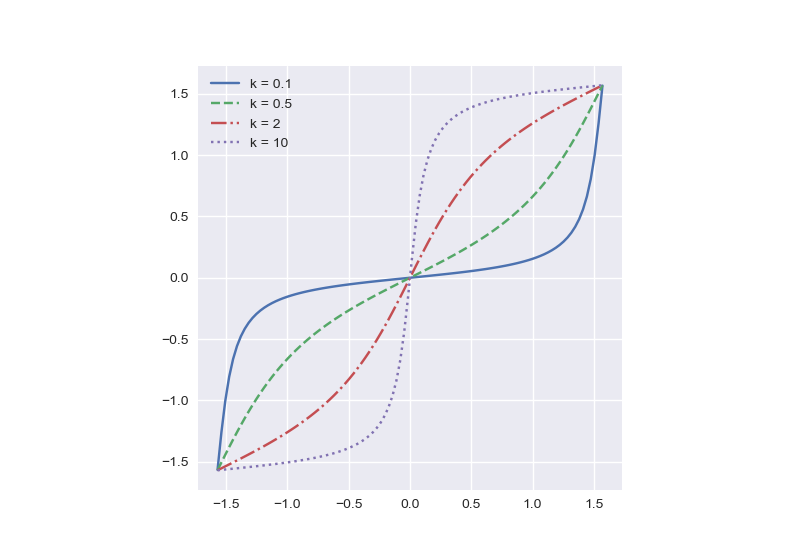# arctan( k tan(x) )

I recently had to work with the function

f(x; k) = arctan( k tan(x) )

The semicolon between x and k is meant to imply that we’re thinking of x as the argument and k as a parameter.

This function is an example of the coming full circle theme I’ve written about several times. Here’s how a novice, a journeyman, and an expert might approach studying our function.

• Novice: arctan( k tan(x) ) = kx.
• Journeyman: You can’t do that!
• Expert: arctan( k tan(x) ) ≈ kx for small x.

Novices often move symbols around without thinking about their meaning, and so someone might pull the k outside (why not?) and notice that arctan( tan(x) ) = x.

Someone with a budding mathematical conscience might conclude that since our function is nonlinear in x and in k that there’s not much that can be said without more work.

Someone with more experience might see that both tan(x) and arctan(x) have the form x + O(x³) and so

arctan( k tan(x) ) ≈ kx

should be a very good approximation for small x.

Here’s a plot of our function for varying values of k.Each is fairly flat in the middle, with slope equal to its value of k.

As k increases, f(x; k) becomes more and more like a step function, equal to -π/2 for negative x and π/2 for positive x, i.e.

arctan( k tan(x) ) ≈ sgn(x) π/2

for large k. Here again we might have discussion like above.

• Novice: Set k = ±∞. Then ±∞ tan(x) = ±∞ and arctan(±∞) = ±π/2.
• Journeyman: You can’t do that!
• Expert: Well, you can if you interpret everything in terms of limits.